Pages

Playing video on nokia color LCD just using an 8 bit AVR! [A mad Project ;) ]

SD CARD + ATMEGA32 + NOKIA COLOR LCD = VIDEO PLAYER!!!
Hi,
I am introducing my new video player made using an atmega32 microcontroller and nokia color LCD. I got a 65K color LCD from an old nokia 6030 mobile phone.  I directly soldered 10 thin enamelled coper wire from the 0.5 cm square area of the thin flexible pcb of the LCD to a berg strip fixed on another board. For me it was the most difficult part of this project because I don't have any tiny tip soldering iron and an lcd connector (5x2) socket with me.
After that I interfaced the lcd with atmega32 via SPI. Then initialized the lcd and displayed some color patterns and confirmed the LCD is working.
Now my next step is to display a still image on the LCD. I used python image library to extract pixel information from any image file (jpeg, png etc) and I streamed it to avr via uart using pyserial and displayed the image successfully on the LCD with 16bit color depth.
After that I used an SD card to store the converted pixel information (132x132*2) bytes/picture. Then I interfaced the SD card with same SPI of atmega32 and displayed the image stored on it. I used FAT16 filesystem. The sd card part is not a problem for me because I have already done more projects on SD/MMC cards and I copied my previous code for that.
So after displaying the still image, I thought of making a slide show on the lcd. For that I wrote a python script to convert all images in a folder (on my pc) to a 132x132x2 byte files which is nothing but the uncompressed pixel information stored as new files. Then I copied all these converted files to SD card (file.lcd) and displayed them as an image slide show with 1 second time gap between each image.
Since I successfully did the image slide show, then suddenly the idea of making a video player came to my mind. Because video is nothing but a slide show at high frame rate.
Then I used ffmpeg to convert a sample video to frames at 15 frames/second & 132x65 resolution. Then I used my python script to convert each still images to pixel information at 16 bit/pixel. Here instead of making small small files, I just collected all the picture information of adjacent frames into a single file and named  as my_video.lcd. Then I copied that file to memory card and modified the avr program to display it on the LCD. It access the FAT16 file system, then search for *.lcd files and if found, it returns the starting cluster address of that file and then stream that cluster to the LCD very fast. NOW VIDEO IS PLAYED (without audio)!!!!! ....Almost half of the project is finished....
Next half: (audio mixing)
I played 15 fps 16bit/pixel on the lcd. Now comes the next headache. Video should have audio. :-(.... Then I started thinking how to include audio. I used ffmpeg(in pc) to extract audio from the video file to 8 bit mono wav. Since wav is uncompressed audio, it is easy to handle it using a microcontroller with hardware PWM. Then I modified my python script. I just opened the wav file and discarded the first 44 bytes (wav header) and then after that, I read the audio sample byte by byte and injected it in between the video information after some calculations(see the math below) related to the bit rate of both audio and video to decide the mixing ratio.
For the perfect audio video synchronization, I implemented an error correction code in the converter, which monitors the synchronization error while mixing the audio-video bytes and when the error reaches a threshold value equivalent, it writes a dummy audio sample instead of real audio sample and thus prevents the accumulation of error and thus perfect audio video synchronization is achieved for hours of continuous video playback..
Now in avr, i need to extract the audio and video and need to send the audio to an audio circular buffer which will be send to PWM using a timer interrupt and video directly to LCD. If any single byte mismatch occurred between the video and audio byte, every thing will get collapsed. I then modified the avr code to do as above and finally I played video with audio! :-)

Video byte rate = 9 frames/second = 9*132*65*2 = 154440 bytes/second
Audio byte rate = 11000 bytes/second
Video sample / audio sample rate = 154440/11000 =  14.04
It means, after every 14 bytes of video, I need to put a byte of audio sample in the final video file.
So, It is fine, but there is a small problem... 14.04 means this .04 error which will accumulate on the way and will affect the audio video synchronization badly, it will be worst in long videos. To prevent this, I need to skip adding a real audio sample after every (1/.04) * 14 = 350 video bytes, when it reaches this value, I need to put a dummy audio sample, may be the previous sample itself. Thus the audio will not overtake the video due to the .04 error and thus audio video synchronization is achieved...

LCD controller:
I believe the LCD which I got from the original nokia phone have Philips PCF8833 controller. Because I did the coding according to that controller specifications. In some example codes, I found we need display invertion command, but in my case don't know why, no need to use display inversion.

Note:
The python script is written for linux OS with python 2.x
AVR C code is written for AVR-GCC compiler in linux.

Photos:

Circuit diagram:
Source code for ATmega32 (avr-gcc)
```/*
ATMEGA32 BASED VIDEO PLAYER on NOKIA 132x132 color LCD
author: Vinod S
email:  vinodstanur at gmail dot com
date:   25/6/2012
homepage:    http://blog.vinu.co.in

compiler: avr-gcc

This program is free software: you can redistribute it and/or modify
the Free Software Foundation, either version 3 of the License, or
(at your option) any later version.

This program is distributed in the hope that it will be useful,
but WITHOUT ANY WARRANTY; without even the implied warranty of
MERCHANTABILITY or FITNESS FOR A PARTICULAR PURPOSE.  See the
GNU General Public License for more details.

You should have received a copy of the GNU General Public License
along with this program.  If not, see <http://www.gnu.org/licenses/>.
*/

#include<avr/io.h>
#define F_CPU 16000000
#include <util/delay.h>
#include <avr/interrupt.h>
#include <string.h>

#define USART_BAUD 115200ul
#define baud (F_CPU/(8*USART_BAUD))-1

#define byte unsigned char

#define RC5_LED PB0
#define SD_CS PB4
#define LCD_CS PB3
#define CHIPSELECT(x) {PORTB |= (1<<LCD_CS)|(1<<SD_CS);PORTB &= ~(1<<x);}
#define SPI_OFF SPCR &= ~(1<<SPE)
#define SPI_ON SPCR |= (1<<SPE)

#define LEFT_SWITCH() ((PIND & (1<<2))==0)
#define RIGHT_SWITCH() ((PIND & (1<3))==0)
#define SWITCH_EVENT() ((PIND & ((1<<2)|(1<<3))) != 0b00001100)
#define SWITCH_ENABLE() do{DDRD &= ~((1<<PD2)|(1<<PD3));PORTD |= ((1<<PD2)|(1<<PD3));} while(0)

void setPixel(unsigned char r, unsigned char g, unsigned char b);
void lcd_init();
void LCD_COMMAND(unsigned char cmd);
void LCD_DATA(unsigned char cmd);
void shiftBits(unsigned char b);
void setPixel(unsigned char r, unsigned char g, unsigned char b);
void uart_send_byte(unsigned char c);
void lcd_init();
void display_black();
void display_pattern();
unsigned int count;
unsigned long int arg = 0;
unsigned char mmc_buf;
unsigned char mmc_audio_buffer;
unsigned int fat_start, dir_start, data_start;
unsigned char sect_per_clust;
unsigned int STARTING_CLUSTER;
void spi_init();
void spi_write(char);
unsigned char command(unsigned char, unsigned long int, unsigned char);
char mmc_init();
void fat16_init();
void print_num(unsigned long int, char);
unsigned int scan_root_dir(unsigned char *, char[], char);
char display_cluster(unsigned int);
unsigned int find_next_cluster(unsigned int);
void pwm_init();
void mmc_read_to_buffer(unsigned long int, unsigned char[]);
void pwm_init();
volatile unsigned char stack = 0, point1 = 0;
volatile char acount = 0;
char NEXT_OR_PREVIOUS = 1;

ISR(TIMER1_COMPA_vect)
{
OCR2 = mmc_audio_buffer[stack++];
}

void timer1_init()
{
TCCR1B |= (1 << WGM12) | (1 << CS10);
TCNT1 = 0;
OCR1A = 1500;
TIMSK |= (1 << OCIE1A);
}

void uart_init()
{
UBRRH = (unsigned char)(baud >> 8);
UBRRL = (unsigned char)baud;
UCSRB = (1 << TXEN) | (1 << RXCIE);
DDRD |= 1 << PD1;
UCSRC = (1 << URSEL) | (3 << UCSZ0);
UCSRA = 1 << U2X;
}

void uart_send(unsigned char c)
{
while (!(UCSRA & (1 << UDRE))) ;
UDR = c;
_delay_ms(1);
}

void string(unsigned char *p, unsigned char c)
{
while (*p)
uart_send(*p++);
}

int main()
{
unsigned char fname;
unsigned int cluster;
spi_init();
CHIPSELECT(LCD_CS);
lcd_init();
timer1_init();
uart_init();
SWITCH_ENABLE();
pwm_init();
_delay_ms(50);
CHIPSELECT(SD_CS);
while (mmc_init()) ;
fat16_init();
while (1) {
while ((cluster =
scan_root_dir("VIN", fname, NEXT_OR_PREVIOUS)) == 0) {
NEXT_OR_PREVIOUS = 1;
}
NEXT_OR_PREVIOUS = 1;
string(fname, 1);

CHIPSELECT(LCD_CS);

display_pattern();
_delay_ms(500);
display_black();

LCD_COMMAND(0x2A);
LCD_DATA(0);
LCD_DATA(131);

LCD_COMMAND(0x2B);
LCD_DATA(33);
LCD_DATA(33 + 64);

LCD_COMMAND(0x2C);
CHIPSELECT(SD_CS);
acount = 0;
sei();
while (cluster != 0xffff) {
if (display_cluster(cluster))
break;
cluster = find_next_cluster(cluster);
}
cli();
if (LEFT_SWITCH())
NEXT_OR_PREVIOUS = 0;
else if (RIGHT_SWITCH())
NEXT_OR_PREVIOUS = 1;
}
return 0;
}

char display_cluster(unsigned int cluster)
{
static unsigned char r, g, b, check = 0;
static unsigned int pixel = 0;
unsigned long int sector;
int i, j;
sector = ((unsigned long int)(cluster - 2) * sect_per_clust);
sector += data_start;
for (i = 0; i < sect_per_clust; i++) {
CHIPSELECT(LCD_CS);
for (j = 0; j < 512; j++) {
if (acount == 14) {
mmc_audio_buffer[point1++] = mmc_buf[j];
acount = 0;
} else {
SPI_OFF;
PORTB &= ~(1 << PB7);
PORTB |= 1 << PB5;
PORTB |= 1 << PB7;
SPI_ON;
SPDR = mmc_buf[j];
if (SWITCH_EVENT()) {
while (!(SPSR & (1 << SPIF))) ;
CHIPSELECT(SD_CS);
return 1;
}
while (!(SPSR & (1 << SPIF))) ;
acount++;
}
}

CHIPSELECT(SD_CS);
sector += 1;
}
return 0;
}

unsigned int find_next_cluster(unsigned int cluster)
{
unsigned int cluster_index_in_buff;
cluster_index_in_buff = (2 * (cluster % 256));
return ((mmc_buf[cluster_index_in_buff + 1] << 8) +
mmc_buf[cluster_index_in_buff]);
}

void mmc_read_to_buffer(unsigned long int sector, unsigned char a[])
{
int i;
sector *= 512;
if (command(17, sector, 0xff) != 0)
for (i = 0; i < 512; i++) {
SPDR = 0xff;
while (!(SPSR & (1 << SPIF))) ;
a[i] = SPDR;
}
spi_write(0xff);
spi_write(0xff);
}

unsigned int scan_root_dir(unsigned char *FILE_EXTENSION, char FNAME[],
char UP_DOWN)
{
while (1) {
unsigned int i;
static unsigned char read_end = 0;
static int base_count = -32, sect_plus = 0;
if (UP_DOWN == 1) {
base_count += 32;
if (base_count == 512) {
base_count = 0;
sect_plus += 1;
};
} else {
base_count -= 32;
if (base_count == -32) {
base_count = (512 - 32);
sect_plus -= 1;
}
if (sect_plus < 0) {
sect_plus = 0;
base_count = 0;
}
}
while (1) {
while (base_count < 512) {
if (mmc_buf[base_count] == 0) {
break;
}
if ((mmc_buf != 0)
&& (mmc_buf[base_count + 2] != 0)
&& (mmc_buf[base_count] != 0xe5)
&& (mmc_buf[base_count] != 0x00)
&& ((mmc_buf[base_count + 11] & 0b00011110)
== 0)
&&
(strncmp
(mmc_buf + base_count + 8, FILE_EXTENSION,
3)
== 0)) {
for (i = 0; i < 11; i++)
FNAME[i] =
mmc_buf[base_count + i];
FNAME = 0;
return (STARTING_CLUSTER =
(unsigned
int)((mmc_buf[27 + base_count]
<< 8)
+ mmc_buf[26 +
base_count]));
}
if (UP_DOWN)
base_count += 32;
else
base_count -= 32;
}
base_count = 0;
sect_plus++;
base_count = -32;
sect_plus = 0;
return 0;
}
}
}
}

void fat16_init()        //BOOT SECTOR SCANNING//
{

if ((mmc_buf[0x36] == 'F') && (mmc_buf[0x39] == '1')
&& (mmc_buf[0x3a] == '6'))
string("FAT16 DETECTED", 1);
else {
string("NOT A FAT16", 1);
while (1) ;
}
_delay_ms(500);
fat_start = mmc_buf[0x0e];
dir_start = (fat_start + (((mmc_buf[0x17] << 8) + mmc_buf[0x16]) * 2));
data_start =
(dir_start +
((((mmc_buf[0x12] << 8) + (mmc_buf[0x11])) * 32) / 512));
sect_per_clust = mmc_buf[0x0d];
}

{
int i;

sector *= 512;
if (command(17, sector, 0xff) != 0)
for (i = 0; i < 512; i++)
spi_write(0xff);
spi_write(0xff);
}

char mmc_init()
{
int u = 0;
unsigned char ocr;
PORTB |= 1 << SD_CS;
for (u = 0; u < 50; u++) {
spi_write(0xff);
}
PORTB &= ~(1 << SD_CS);
_delay_ms(1);
count = 0;
while (command(0, 0, 0x95) != 1 && (count++ < 1000)) ;
if (count > 900) {
string("CARD ERROR-CMD0 ", 1);
_delay_ms(500);
return 1;
}
if (command(8, 0x1AA, 0x87) == 1) {    /* SDC ver 2.00 */
for (u = 0; u < 4; u++)
if (ocr == 0x01 && ocr == 0xAA) {    /* The card can work at vdd range of 2.7-3.6V */
count = 0;
do
command(55, 0, 0xff);
while (command(41, 1UL << 30, 0xff) && count++ < 1000);    /* ACMD41 with HCS bit */
if (count > 900) {
string("ERROR SDHC 41", 1);
return 1;
}
count = 0;
if (command(58, 0, 0xff) == 0 && count++ < 1000) {    /* Check CCS bit */
for (u = 0; u < 4; u++)
}
}
} else {
command(55, 0, 0xff);
if (command(41, 0, 0xff) > 1) {
count = 0;
while ((command(1, 0, 0xff) != 0) && (count++ < 1000)) ;
if (count > 900) {
string("CARD ERROR-CMD1 ", 1);
_delay_ms(500);
return 1;
}
} else {
count = 0;
do {
command(55, 0, 0xff);
}
while (command(41, 0, 0xff) != 0 && count < 1000);
}

if (command(16, 512, 0xff) != 0) {
string("CARD ERROR-CMD16 ", 1);
_delay_ms(500);
return 1;
}
}
string("MMC INITIALIZED!", 1);
_delay_ms(500);
SPCR &= ~(1 << SPR1);    //increase SPI clock from f/32 to f/2
return 0;
}

unsigned char command(unsigned char command,
unsigned long int fourbyte_arg, unsigned char CRCbits)
{
unsigned char retvalue, n;
spi_write(0xff);
spi_write(0b01000000 | command);
spi_write((unsigned char)(fourbyte_arg >> 24));
spi_write((unsigned char)(fourbyte_arg >> 16));
spi_write((unsigned char)(fourbyte_arg >> 8));
spi_write((unsigned char)fourbyte_arg);
spi_write(CRCbits);
n = 10;
do
while ((retvalue & 0x80) && --n);

return retvalue;
}

{
SPDR = 0xff;
while (!(SPSR & (1 << SPIF))) ;
return SPDR;
}

void spi_write(char cData)
{
SPDR = cData;
while (!(SPSR & (1 << SPIF))) ;
}

void spi_init()
{
PORTC = 0;
PORTB = (1 << PB4) | (1 << PB3);
DDRC |= 1 << PC4;
DDRB |= (1 << 5) | (1 << 7) | (1 << 4) | (1 << 3);
_delay_ms(10);
PORTB |= (1 << SD_CS) | (1 << LCD_CS);
SPCR =
(1 << SPE) | (1 << MSTR) | (1 << SPR1) | (1 << CPHA) | (1 << CPOL);
SPSR = 1;
}

void lcd_init()
{

SPI_OFF;

PORTB &= ~(1 << PB7);
PORTB &= ~(1 << PB5);
PORTB |= 1 << PB7;

//RESET
PORTC |= 1 << PC4;
PORTC &= ~(1 << PC4);
PORTC |= 1 << PC4;
PORTC &= ~(1 << PC4);
PORTC |= 1 << PC4;
PORTC &= ~(1 << PC4);
PORTC |= 1 << PC4;

SPI_ON;

LCD_COMMAND(0x01);

//Sleep Out
LCD_COMMAND(0x11);

//Booster ON
LCD_COMMAND(0x02);

_delay_ms(10);

//Display On
LCD_COMMAND(0x29);

//Normal display mode
// LCD_COMMAND(0x13);

//Display inversion on
//LCD_COMMAND(0x21);

//Data order
LCD_COMMAND(0xBA);

//Memory data access control
LCD_COMMAND(0x36);

//LCD_DATA(8|64);   //rgb + MirrorX
LCD_DATA(8 | 128);    //rgb + MirrorY

LCD_COMMAND(0x3A);
LCD_DATA(5);        //16-Bit per Pixel

//Set Constrast
LCD_COMMAND(10);
LCD_DATA(50);
display_black();

}

void display_black()
{
int i, j;
for (i = -66; i < 66; i++) {
for (j = -66; j < 66; j++) {
setPixel(0, 0, 0);
}
}
}

void display_pattern()
{
LCD_COMMAND(0x2A);
LCD_DATA(0);
LCD_DATA(131);

LCD_COMMAND(0x2B);
LCD_DATA(0);
LCD_DATA(131);

//Memory Write

LCD_COMMAND(0x2C);
int16_t i, j;
int32_t p;

for (i = -66; i < 66; i++) {
for (j = -66; j < 66; j++) {
p = ((i) * (i)) + ((j) * (j));
if (p >= 2000 && p < 3000)
setPixel(255, 0, 0);
else if (p >= 1000 && p < 2000)
setPixel(0, 255, 0);
else if (p < 1000)
setPixel(0, 0, 255);
else if (i % 5 == 0)
setPixel(255, 255, 255);
else
setPixel(0, 0, 0);
}
}
}

//send data
void LCD_DATA(byte data)
{

SPI_OFF;
PORTB &= ~(1 << PB7);
PORTB |= 1 << PB5;
PORTB |= 1 << PB7;
SPI_ON;
SPDR = data;
while (!(SPSR & (1 << SPIF))) ;
}

void LCD_COMMAND(byte data)
{
SPI_OFF;
PORTB &= ~(1 << PB7);
PORTB &= ~(1 << PB5);
PORTB |= 1 << PB7;
SPI_ON;
SPDR = data;
while (!(SPSR & (1 << SPIF))) ;
}

void setPixel(byte r, byte g, byte b)
{
LCD_DATA((b & 248) | g >> 5);
LCD_DATA(((g << 3) & 0b11100000) | r >> 3);
}

void pwm_init()
{
TCCR2 |= (1 << WGM20) | (1 << WGM21) | (1 << COM21) | (1 << CS20);
DDRD |= (1 << PD7);
}
```

VIDEO CONVERTER PROGRAM:(python script)
(to be saved as converter.py)
(Python image library and ffmpeg should be installed before running this script)
FOR LINUX ONLY

```############################################################
# video converter software to convert any video to         #
# .vin format, (special format for the avr video player    #
# author: Vinod S                                          #
#         vinodstanur at gmail dot com                     #
#home:  http://blog.vinu.co.in                             #
#USAGE:                                                    #
# python converter.py input_file_name seek_time duration   #
# example: python converter.py a.avi 0:05:00 00:01:00      #
# above code will convert a.avi from time = 5 minutes to   #
#  6 minutes                                               #
############################################################

#!/usr/bin/python
import time,sys,os,Image
time = sys.argv
seek = sys.argv
videofile = sys.argv
videofile = videofile.replace(" ","\ ")
videofile = videofile.replace("(","\(")
videofile = videofile.replace(")","\)")
os.system("clear")
count = 1
os.system("rm clip.avi")
os.system("rm *.jpeg audio.wav")
os.system("ffmpeg -ss " + seek + " -t " + time + " -y -i " + videofile + " -acodec libmp3lame -vcodec msmpeg4 -ab 8k -b 1000k -vf scale=132:65 -ar 8000 -r:v 9 clip.avi")
os.system("ffmpeg -i clip.avi %d.jpeg")
os.system("ffmpeg -i "+ videofile + " -ac 1 -acodec pcm_u8 -ac 1 -ar 11000 -t "+ time + " -ss "+ seek  + " -vn audio.wav")
files = os.listdir(".")
afd = open("audio.wav")
a=0
b=0
total_jpeg = 0
for i in files:
if i[-4:]=="jpeg":
total_jpeg =total_jpeg+ 1
total_jpeg = total_jpeg-1
print str(total_jpeg)
f=open("out.vin","w")
acount=0
skip = 0
while count < total_jpeg:
print str(count)
v = Image.open(str(count)+".jpeg").convert("RGB")
for i in range(65):
for j in range(132):
if acount==7:
if skip == 25*14:
skip = 0
else:
skip = skip + 1
acount = 0
acount = acount + 1
tup = v.getpixel((131-j,i))
a=(tup&248)|(tup>>5)
b=((tup<<3)&224)|(tup>>3)
f.write(chr(a))
f.write(chr(b))
count = count + 1
del v
f.close()
os.system("rm clip.avi")
os.system("rm *.jpeg")
os.system("rm audio.wav") ```

1.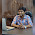great work brother....you are really amazing as your projects....hey help me, i am a new be to microcontroller, so how can i do such type of project of my own...???thanks a lot for your tutorials...

2.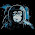whew.. iam amazed!

These cheap little 8BIT AVR`s surprise me again and again to the new :)

3.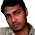@Satya Sankar: Hi,
"so how can i do such type of project of my own?"

If you are a beginner to microcontroller, you have to practice some basic programs and need to get familiarized with few modules like SPI, UART, PWM etc. After that you can try this type of projects. That is what I do....

4.postingan yang bagus...........

5.mast hai bhai bharat mata ki jai !!!!!

6.Brother really amazing

7.very good project and amazing work!!!
I am also trying to implement color LCD interfacing with atmega64 but i thought SRAM iil be the problem but u did great job..!!

8.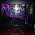Man that breadboard looks like its on life support LOL.

- http://custompcstuff.blogspot.com/

9.YOU ARE GENIUS!

10.Mr vinod could we have a hand some lcd backlight circiut for the lcd or would an external dc supply + 7v adjustable by a diodebank in series(drops .7v each) coud do the job ,is there a bit of sophistication out there like using PWM routines ,or is it only in case of contrast controll . (joseandjust@gmail.com)

11.u hav'nt mentioned the chipselect connection in your diagram,would this mean you made some other pin to routine the SPI signals.....for the mmc and lcd,also please mention in your schematic the role of PB3,PC4,the second MOSI and SCK linking the LCD

12.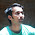Sir I am Confused why you use "PYTHON" Scripting Language for ATMEGA32 Microcontroller I mean if I am not wrong then to program "Microcontroller " we need .asm or .c code and not the phython..............please help

13.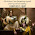sir
where did you connect pin 7 of lcd

14.First off awesome movie choice. Secondly do you know how challenging it would be to use a different lcd? One that is bigger and higher resolution?

15.This is one the best blogs i have ever come across on the internet. Have been reading posts from your blog, really interesting. Independence Day Speech for Teachers